# Half-life

If a sample of material decays at a certain rate over time, its half-life is defined as the time it takes for the sample to decay to half its initial amount. This concept originated when studying the exponential decay of radioactive isotopes, but it is applied to other phenomena as well, including those described by non-exponential decay. An exponential decay process, as exemplified by the decay of radioactive isotopes, for example, is simply one in which the number of atoms disintegrating per unit time is proportional to the total number of radioactive atoms present.

In the case of radioactive decay, each different radioactive isotope has a particular half-life that is unaffected by changes in the physical or chemical conditions of the surroundings. This property is the basis for radiometric dating of rocks and fossils. In pharmacology, the half-life of a drug (in a biological system) is the time it takes for the drug to lose half its pharmacologic activity.

Carbon-14 (14C) is a radioactive isotope that decays to produce the isotope nitrogen-14 (14N). The half-life of 14C is about 5,730 years. This means that if one starts with 10 grams of 14C, then 5 grams of the isotope will remain after 5,730 years, 2.5 grams will remain after another 5,730 years, and so forth.

## Calculation of half-life

Number of
half-lives
elapsed
Fraction
remaining
As
power
of 2
0 1/1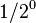$1/2^0$
1 1/2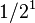$1/2^1$
2 1/4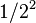$1/2^2$
3 1/8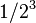$1/2^3$
4 1/16$1/2^4$
5 1/32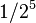$1/2^5$
6 1/64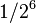$1/2^6$
7 1/128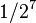$1/2^7$
... ...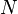$N$$1/2^N$$1/2^N$

The table at right shows the reduction of the quantity in terms of the number of half-lives elapsed.

It can be shown that, for exponential decay, the half-life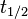$t_{1/2}$ obeys the following relation:$t_{1/2} = \frac{\ln (2)}{\lambda}$

where

•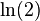$\ln (2)$ is the natural logarithm of 2, and
•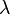$\lambda$, called the decay constant, is a positive constant used to describe the rate of exponential decay.

In addition, the half-life is related to the mean lifetime τ by the following relation: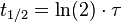$t_{1/2} = \ln (2) \cdot \tau$

The constant$\lambda$ can represent various specific physical quantities, depending on the process being described.

• In first-order chemical reactions,$\lambda$ is the reaction rate constant.
• In pharmacology (specifically pharmacokinetics), the half-life of a drug is defined as the time it takes for a substance (drug, radioactive nuclide, or other) to lose half of its pharmacologic, physiologic, or radiologic activity.
• For electronic filters such as an RC circuit (resistor-capacitor circuit) or an RL circuit (resistor-inductor circuit),$\lambda$ is the reciprocal of the circuit's time constant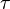$\tau$, which is the same as the mean lifetime mentioned above. For simple RC or RL circuits,$\lambda$ equals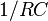$1/RC$ or$R/L$, respectively. The symbol$\tau$ is related to the circuit's cutoff frequency fc by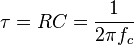$\tau = RC = \frac{1}{2 \pi f_c}$ or, equivalently,$f_c = \frac{1}{2 \pi R C} = \frac{1}{2 \pi \tau}$.

## Experimental determination

The half-life of a process can be readily determined by experiment. Some methods do not require advance knowledge of the law governing the decay rate, whether it follows an exponential or other pattern of decay.

Most appropriate to validate the concept of half-life for radioactive decay, in particular when dealing with a small number of atoms, is to perform experiments and correct computer simulations. Validation of physics-math models consists of comparing the model's behavior with experimental observations of real physical systems or valid simulations (physical and/or computer simulations).

When studying radioactive decay, the exponential model does not apply for a small number of atoms (or a small number of atoms is not within the domain of validity of the formula or equation or table). Some model simulations use pennies or pieces of candy. A similar experiment is performed with isotopes that have very short half-lives.

## Decay by two or more processes

Some quantities decay by two processes simultaneously. In a manner similar to that mentioned above, one can calculate the new total half-life (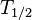$T_{1/2}$) as follows: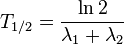$T_{1/2} = \frac{\ln 2}{\lambda _1 + \lambda _2} \,$

or, in terms of the two half-lives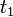$t_1$ and$t_2$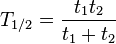$T_{1/2} = \frac{t _1 t _2}{t _1 + t_2} \,$

that is, half their harmonic mean.

## Derivation

Quantities that are subject to exponential decay are commonly denoted by the symbol$N$. (This convention suggests a decaying number of discrete items, an interpretation that is valid in many, but not all, cases of exponential decay.) If the quantity is denoted by the symbol$N$, the value of$N$ at a time$t$ is given by the formula:$N(t) = N_0 e^{-\lambda t} \,$

where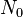$N_0$ is the initial value of$N$ (at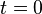$t = 0$)

When$t = 0$, the exponential is equal to 1, and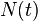$N(t)$ is equal to$N_0$. As$t$ approaches infinity, the exponential approaches zero. In particular, there is a time$t_{1/2} \,$ such that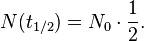$N(t_{1/2}) = N_0\cdot\frac{1}{2}.$

Substituting into the formula above, we have$N_0\cdot\frac{1}{2} = N_0 e^{-\lambda t_{1/2}}, \,$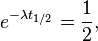$e^{-\lambda t_{1/2}} = \frac{1}{2}, \,$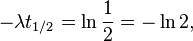$- \lambda t_{1/2} = \ln \frac{1}{2} = - \ln{2}, \,$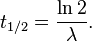$t_{1/2} = \frac{\ln 2}{\lambda}. \,$